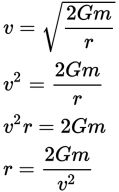# SAT Math Multiple Choice Question 579: Answer and Explanation

### Test Information

Question: 579

9. Escape velocity is the speed that a traveling object needs to break free of a planet or moon's gravitational field without additional propulsion (for example, without using fuel). The formula used to calculate escape velocity is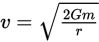, where G represents the universal gravitational constant, m is the mass of the body from which the object is escaping, and r is the distance between the object and the body's center of gravity. Which equation represents the value of r in terms of v, G, and m?

• A.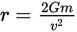• B.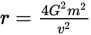• C.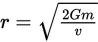• D.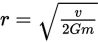Explanation:

A

Difficulty: Medium

Category: Passport to Advanced Math / Exponents

Strategic Advice: Don't spend too much time reading the scientific explanation of the equation. Focus on the question at the very end—it's just asking you to solve the equation for r, or in other words to get r on one side of the equation by itself.

Getting to the Answer: Solve for r using inverse operations. First, square both sides of the equation to remove the radical. Then, multiply both sides by r to get the r out of the denominator. Finally, divide both sides by v2.